|

# 用爱筑造家,43㎡小屋就够了!

这是一个关于爱的故事，一个姑娘通过改造一个43㎡的家为癌症晚期的母亲改善生活环境，原来良好的居住环境和充满爱的氛围真的可以使人开心，连母亲的病似乎也好转了！

妈妈说

我自己知道，身体不好，活一天赚一天……但现在，因为这个美好的家，我又重新燃起了生活的新希望！改造前

改造前的房屋整体就是杂乱无须，生活使用上也很不方面。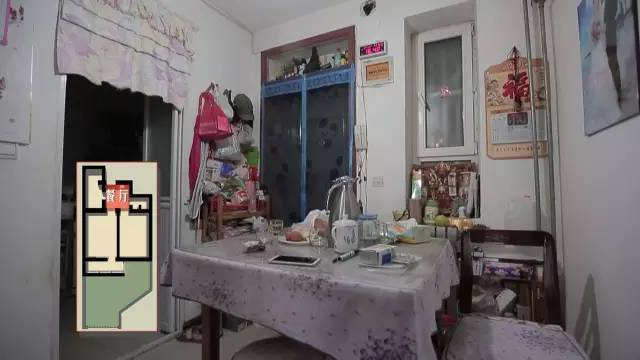餐厅就紧靠着入户门，狭窄拘束，是通向其它居室的必经之地。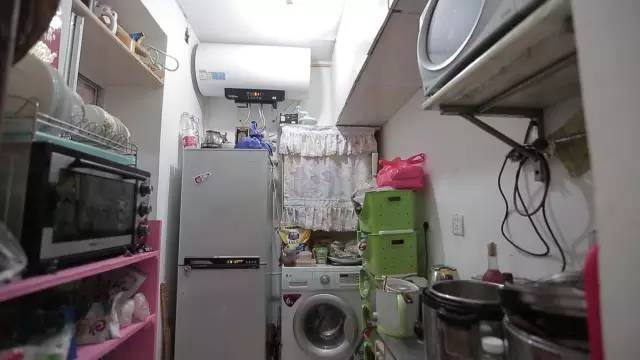厨房既拥挤又潮湿，由于没有排水口，每次洗衣服时都要用软管把洗衣机里的水导到厕所排放。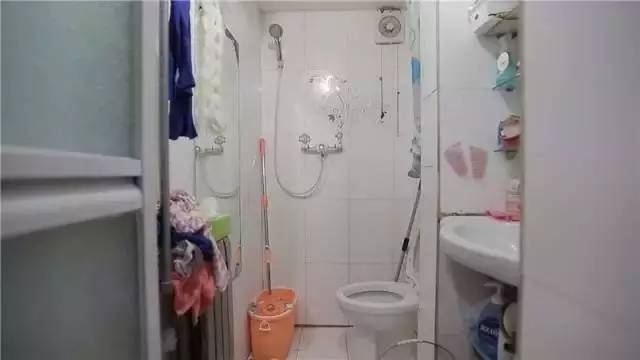卫生间只有2㎡

顶棚低矮采暖差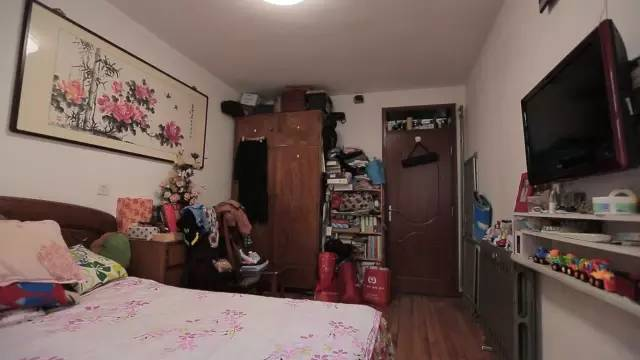每个周末女主人公夏小姐带儿子回家探望，睡觉只能和母亲挤在一张床上的时候，儿子午夜的哭闹总会干扰到母亲的休息，影响母亲的病情。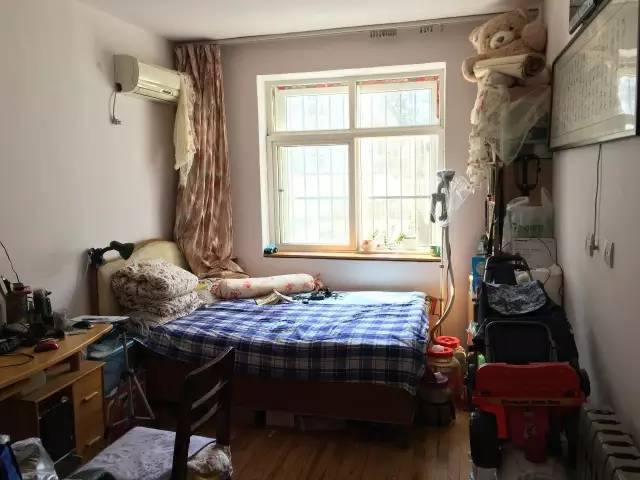次卧是热衷于鼓捣电器的父亲睡觉的地方，堆满了各种工具和杂物。除了43㎡的屋子，从主卧阳台出来是夏小姐家25㎡的院子。院子与房间地面存在近60公分的落差，台阶下面水泥砌成的洞则成了家里小狗哈利的小窝。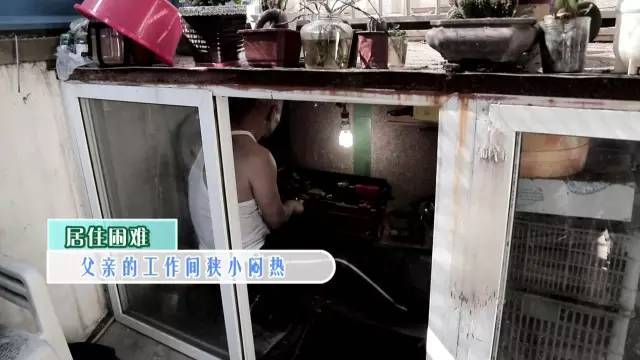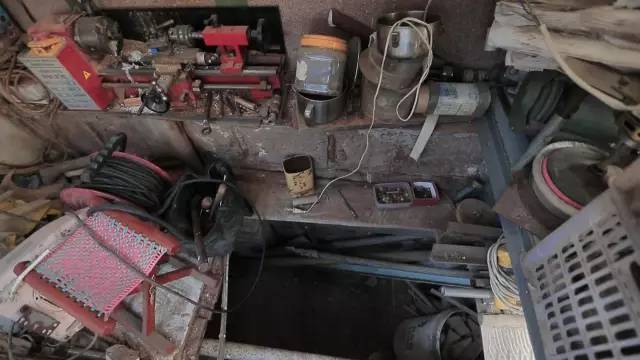院子里地窖改成的工作间

空间狭小闷热

父亲的块头挤在里面工作十分不便

改造方案

改造前的平面图：母亲与父亲分开睡，两个卧室占去了屋子的绝大部分面积，其它功能区过于狭窄，生活十分不便。外面的院子也堆满了杂物，显得十分杂乱。

改造后的平面图：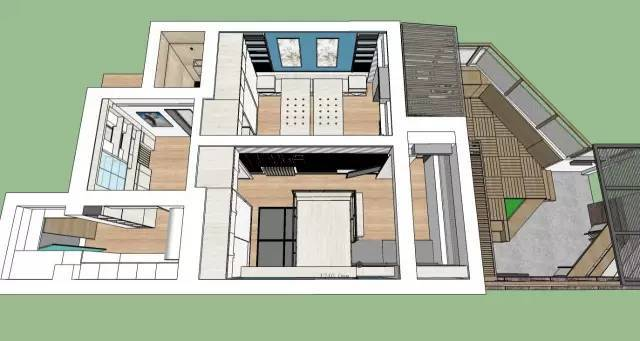入户门改在厨房一侧，原本父亲睡觉的地方被改造成了两张单人床，原来的主卧则变成了集书房、客厅、卧室于一体的空间。外面的院子也重新修葺，打造出工作和休闲的露天花园。

改造后

由于女主人公的母亲身患重病，对于装修的环保性和安全性十分高，所以设计师动用了很多木质元素及最环保的材料，打造了一个由里及外的生机小家！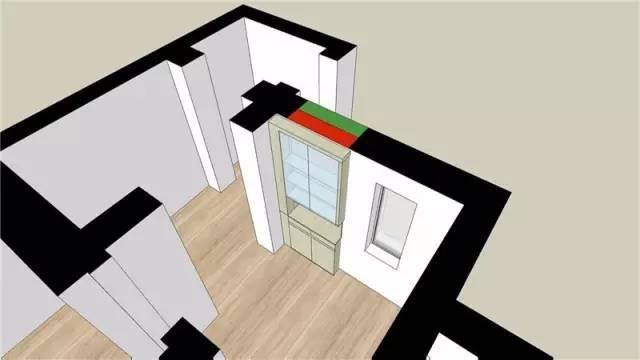原本直通餐区的房门此时已经变成了双面柜。屋外是顶天立地的鞋柜，新的入户门则直接通向了厨房。

厨房▼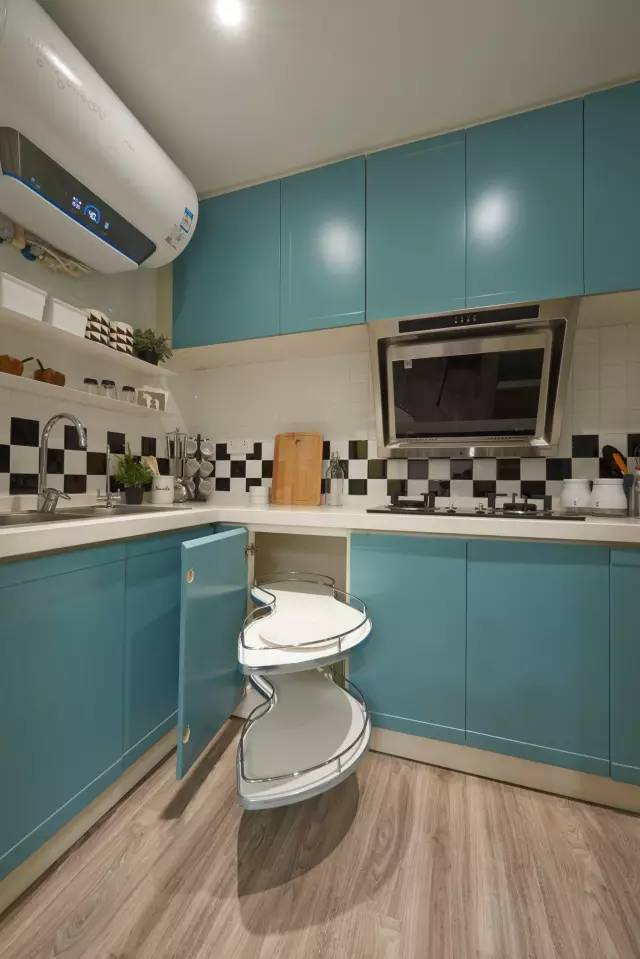厨房的模样焕然一新，湖蓝色的橱柜、黑白相间的墙砖腰线，还有自然系的地板进厨房，看起来很清新时尚。利用夹缝空间设计的高深拉篮

正好弥补了冰箱边空出来的空间

餐厅▼双面柜的另一侧则是餐厅的展示柜，和厨房一样的大面积地板给你以温暖的感觉……

卫生间▼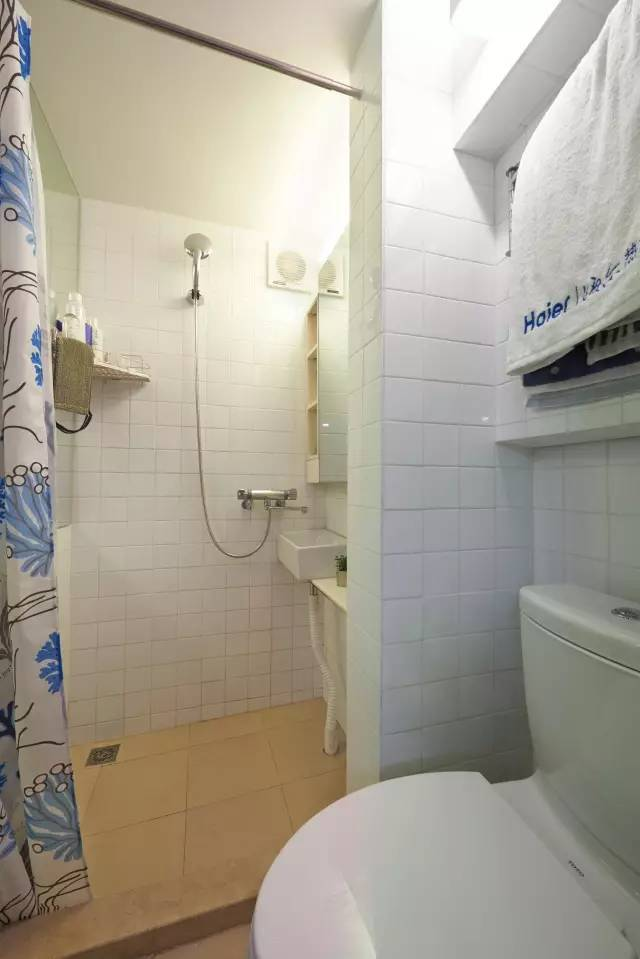原本的洗手池位置变成了现在的坐便器，干湿分离的设计大大提高了父母洗浴时的便捷性。

主卧▼主卧与阳台之间的隔断打通，安装了大大的落地窗，提高了屋内的亮度。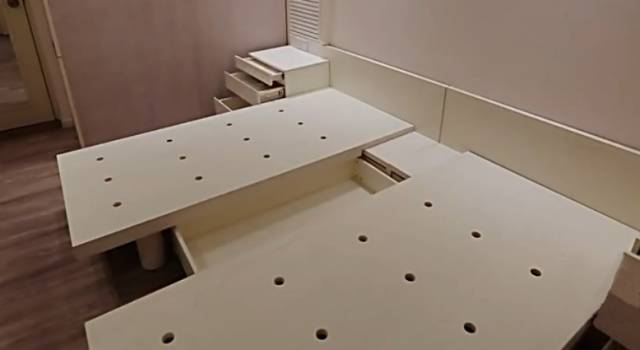为了保持原本父母的睡眠习惯，大床可以按照需要分成两个单人床，这样父亲修理好电器回房的时候也不会打扰到母亲的休息。除了床边的壁柜，

床底和床头还设计了许多收纳空间。

次卧▼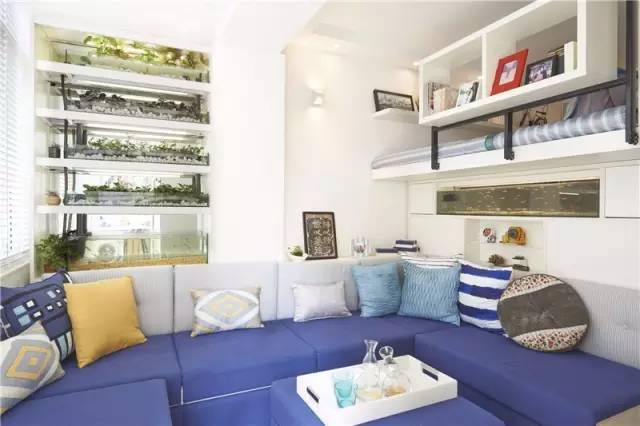次卧被分隔成客厅和书房两个区域。客厅蓝色沙发可以拼成一张可供休息的沙发床。沙发后面的墙面则安装了鱼菜共生装置，给屋子带来绿意与生机。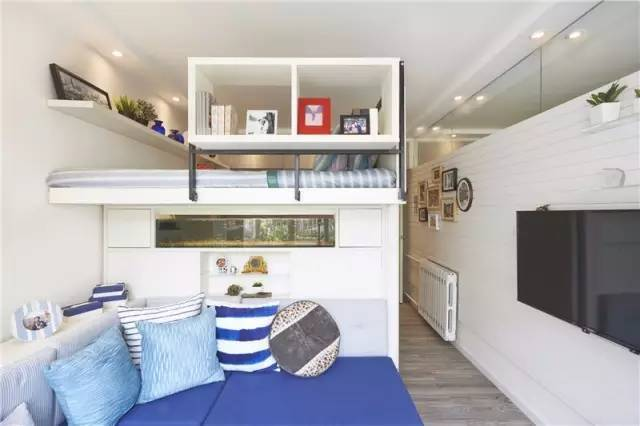沙发与书房隔断中间增加了可观赏鱼缸，

灵动的小鱼给家带来更多活力。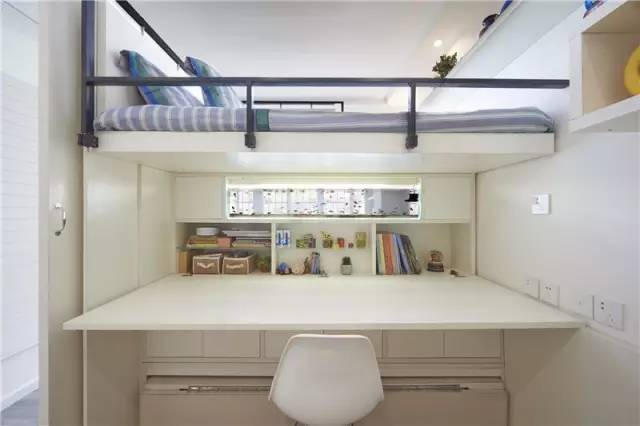多功能家具的变化，

让这里既可以作书房，也可作为卧室。以后夏小姐带着儿子探望母亲的时候，既可以睡在隔断上方的床，也能睡在工作台下面的抽拉床，一边的房门还能保证一定的隐私。

院子▼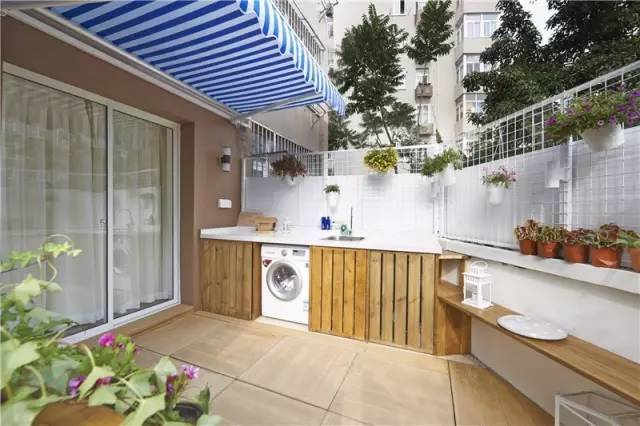主卧的阳台与院子连在一起，原本的60公分高度差被合金框架补平。洗衣机也从原来的厨房搬进了院子，上面的雨棚设计考虑到了遮雨的需要。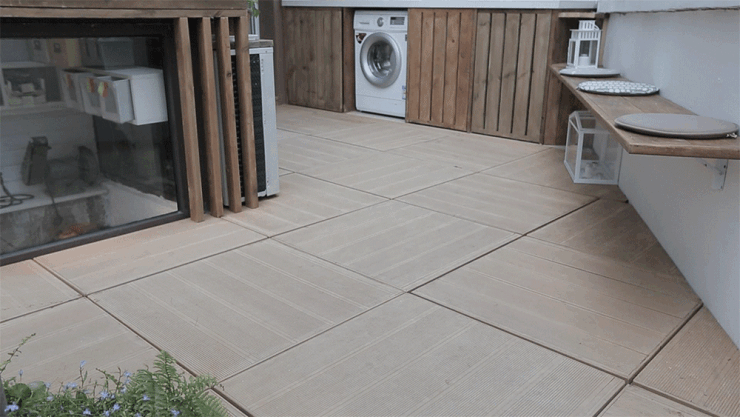垫高的地面下放上防潮箱，就可以用作储纳，

父亲的工具杂物再也不怕没处放了！作为家中的主要入口，

南面的小院被打造成了极为舒适的露天庭院！利用花园的高低落差设置的座位，

配上可变化的桌椅，

成为一个非常舒适的休闲角落。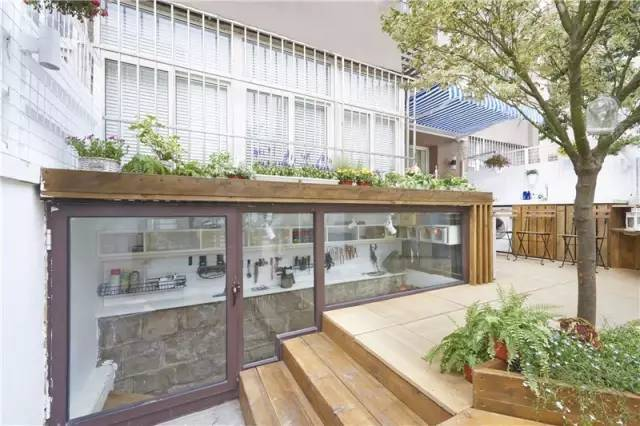还记得父亲原本潮湿拥挤的工作间吗？改造后的工作间，不仅高度舒适，

便于储纳，还设计了可变化的透光百叶顶棚！住在老台阶下面的小狗哈利，

也有了一个舒适的新窝。

最后，再让我们看一下设计师用爱为这对母女改造的成果吧！

厨房▼餐厅▼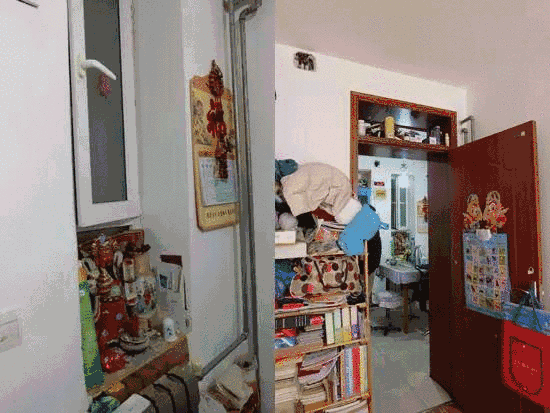重病母亲卧房▼父亲卧室▼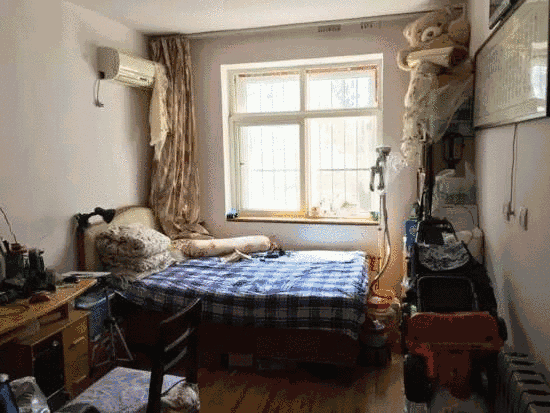屋外院子▼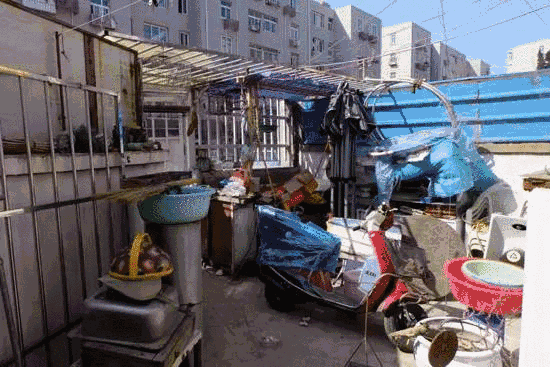这个改造旧屋的过程，让我们看到原来屋虽小但也可以用爱筑造温馨。设计师和近百人一起努力只为给一位母亲一份惊喜，用充满爱的设计打造出重焕光彩的生机小宅！

`声明：本文由入驻焦点开放平台的作者撰写，除焦点官方账号外，观点仅代表作者本人，不代表焦点立场错误信息举报电话： 400-099-0099，邮箱：jubao@vip.sohu.com，或点此进行意见反馈，或点此进行举报投诉。`A B C D E F G H J K L M N P Q R S T W X Y Z
A - B - C - D - E
• A
• 鞍山
• 安庆
• 安阳
• 安顺
• 安康
• 澳门
• B
• 北京
• 保定
• 包头
• 巴彦淖尔
• 本溪
• 蚌埠
• 亳州
• 滨州
• 北海
• 百色
• 巴中
• 毕节
• 保山
• 宝鸡
• 白银
• 巴州
• C
• 承德
• 沧州
• 长治
• 赤峰
• 朝阳
• 长春
• 常州
• 滁州
• 池州
• 长沙
• 常德
• 郴州
• 潮州
• 崇左
• 重庆
• 成都
• 楚雄
• 昌都
• 慈溪
• 常熟
• D
• 大同
• 大连
• 丹东
• 大庆
• 东营
• 德州
• 东莞
• 德阳
• 达州
• 大理
• 德宏
• 定西
• 儋州
• 东平
• E
• 鄂尔多斯
• 鄂州
• 恩施
F - G - H - I - J
• F
• 抚顺
• 阜新
• 阜阳
• 福州
• 抚州
• 佛山
• 防城港
• G
• 赣州
• 广州
• 桂林
• 贵港
• 广元
• 广安
• 贵阳
• 固原
• H
• 邯郸
• 衡水
• 呼和浩特
• 呼伦贝尔
• 葫芦岛
• 哈尔滨
• 黑河
• 淮安
• 杭州
• 湖州
• 合肥
• 淮南
• 淮北
• 黄山
• 菏泽
• 鹤壁
• 黄石
• 黄冈
• 衡阳
• 怀化
• 惠州
• 河源
• 贺州
• 河池
• 海口
• 红河
• 汉中
• 海东
• 怀来
• I
• J
• 晋中
• 锦州
• 吉林
• 鸡西
• 佳木斯
• 嘉兴
• 金华
• 景德镇
• 九江
• 吉安
• 济南
• 济宁
• 焦作
• 荆门
• 荆州
• 江门
• 揭阳
• 金昌
• 酒泉
• 嘉峪关
K - L - M - N - P
• K
• 开封
• 昆明
• 昆山
• L
• 廊坊
• 临汾
• 辽阳
• 连云港
• 丽水
• 六安
• 龙岩
• 莱芜
• 临沂
• 聊城
• 洛阳
• 漯河
• 娄底
• 柳州
• 来宾
• 泸州
• 乐山
• 六盘水
• 丽江
• 临沧
• 拉萨
• 林芝
• 兰州
• 陇南
• M
• 牡丹江
• 马鞍山
• 茂名
• 梅州
• 绵阳
• 眉山
• N
• 南京
• 南通
• 宁波
• 南平
• 宁德
• 南昌
• 南阳
• 南宁
• 内江
• 南充
• P
• 盘锦
• 莆田
• 平顶山
• 濮阳
• 攀枝花
• 普洱
• 平凉
Q - R - S - T - W
• Q
• 秦皇岛
• 齐齐哈尔
• 衢州
• 泉州
• 青岛
• 清远
• 钦州
• 黔南
• 曲靖
• 庆阳
• R
• 日照
• 日喀则
• S
• 石家庄
• 沈阳
• 双鸭山
• 绥化
• 上海
• 苏州
• 宿迁
• 绍兴
• 宿州
• 三明
• 上饶
• 三门峡
• 商丘
• 十堰
• 随州
• 邵阳
• 韶关
• 深圳
• 汕头
• 汕尾
• 三亚
• 三沙
• 遂宁
• 山南
• 商洛
• 石嘴山
• T
• 天津
• 唐山
• 太原
• 通辽
• 铁岭
• 泰州
• 台州
• 铜陵
• 泰安
• 铜仁
• 铜川
• 天水
• 天门
• W
• 乌海
• 乌兰察布
• 无锡
• 温州
• 芜湖
• 潍坊
• 威海
• 武汉
• 梧州
• 渭南
• 武威
• 吴忠
• 乌鲁木齐
X - Y - Z
• X
• 邢台
• 徐州
• 宣城
• 厦门
• 新乡
• 许昌
• 信阳
• 襄阳
• 孝感
• 咸宁
• 湘潭
• 湘西
• 西双版纳
• 西安
• 咸阳
• 西宁
• 仙桃
• 西昌
• Y
• 运城
• 营口
• 盐城
• 扬州
• 鹰潭
• 宜春
• 烟台
• 宜昌
• 岳阳
• 益阳
• 永州
• 阳江
• 云浮
• 玉林
• 宜宾
• 雅安
• 玉溪
• 延安
• 榆林
• 银川
• Z
• 张家口
• 镇江
• 舟山
• 漳州
• 淄博
• 枣庄
• 郑州
• 周口
• 驻马店
• 株洲
• 张家界
• 珠海
• 湛江
• 肇庆
• 中山
• 自贡
• 资阳
• 遵义
• 昭通
• 张掖
• 中卫

1室1厅1厨1卫1阳台

1
2
3
4
5

0
1
2

1

1

0
1
2
3报名成功，资料已提交审核A B C D E F G H J K L M N P Q R S T W X Y Z
A - B - C - D - E
• A
• 鞍山
• 安庆
• 安阳
• 安顺
• 安康
• 澳门
• B
• 北京
• 保定
• 包头
• 巴彦淖尔
• 本溪
• 蚌埠
• 亳州
• 滨州
• 北海
• 百色
• 巴中
• 毕节
• 保山
• 宝鸡
• 白银
• 巴州
• C
• 承德
• 沧州
• 长治
• 赤峰
• 朝阳
• 长春
• 常州
• 滁州
• 池州
• 长沙
• 常德
• 郴州
• 潮州
• 崇左
• 重庆
• 成都
• 楚雄
• 昌都
• 慈溪
• 常熟
• D
• 大同
• 大连
• 丹东
• 大庆
• 东营
• 德州
• 东莞
• 德阳
• 达州
• 大理
• 德宏
• 定西
• 儋州
• 东平
• E
• 鄂尔多斯
• 鄂州
• 恩施
F - G - H - I - J
• F
• 抚顺
• 阜新
• 阜阳
• 福州
• 抚州
• 佛山
• 防城港
• G
• 赣州
• 广州
• 桂林
• 贵港
• 广元
• 广安
• 贵阳
• 固原
• H
• 邯郸
• 衡水
• 呼和浩特
• 呼伦贝尔
• 葫芦岛
• 哈尔滨
• 黑河
• 淮安
• 杭州
• 湖州
• 合肥
• 淮南
• 淮北
• 黄山
• 菏泽
• 鹤壁
• 黄石
• 黄冈
• 衡阳
• 怀化
• 惠州
• 河源
• 贺州
• 河池
• 海口
• 红河
• 汉中
• 海东
• 怀来
• I
• J
• 晋中
• 锦州
• 吉林
• 鸡西
• 佳木斯
• 嘉兴
• 金华
• 景德镇
• 九江
• 吉安
• 济南
• 济宁
• 焦作
• 荆门
• 荆州
• 江门
• 揭阳
• 金昌
• 酒泉
• 嘉峪关
K - L - M - N - P
• K
• 开封
• 昆明
• 昆山
• L
• 廊坊
• 临汾
• 辽阳
• 连云港
• 丽水
• 六安
• 龙岩
• 莱芜
• 临沂
• 聊城
• 洛阳
• 漯河
• 娄底
• 柳州
• 来宾
• 泸州
• 乐山
• 六盘水
• 丽江
• 临沧
• 拉萨
• 林芝
• 兰州
• 陇南
• M
• 牡丹江
• 马鞍山
• 茂名
• 梅州
• 绵阳
• 眉山
• N
• 南京
• 南通
• 宁波
• 南平
• 宁德
• 南昌
• 南阳
• 南宁
• 内江
• 南充
• P
• 盘锦
• 莆田
• 平顶山
• 濮阳
• 攀枝花
• 普洱
• 平凉
Q - R - S - T - W
• Q
• 秦皇岛
• 齐齐哈尔
• 衢州
• 泉州
• 青岛
• 清远
• 钦州
• 黔南
• 曲靖
• 庆阳
• R
• 日照
• 日喀则
• S
• 石家庄
• 沈阳
• 双鸭山
• 绥化
• 上海
• 苏州
• 宿迁
• 绍兴
• 宿州
• 三明
• 上饶
• 三门峡
• 商丘
• 十堰
• 随州
• 邵阳
• 韶关
• 深圳
• 汕头
• 汕尾
• 三亚
• 三沙
• 遂宁
• 山南
• 商洛
• 石嘴山
• T
• 天津
• 唐山
• 太原
• 通辽
• 铁岭
• 泰州
• 台州
• 铜陵
• 泰安
• 铜仁
• 铜川
• 天水
• 天门
• W
• 乌海
• 乌兰察布
• 无锡
• 温州
• 芜湖
• 潍坊
• 威海
• 武汉
• 梧州
• 渭南
• 武威
• 吴忠
• 乌鲁木齐
X - Y - Z
• X
• 邢台
• 徐州
• 宣城
• 厦门
• 新乡
• 许昌
• 信阳
• 襄阳
• 孝感
• 咸宁
• 湘潭
• 湘西
• 西双版纳
• 西安
• 咸阳
• 西宁
• 仙桃
• 西昌
• Y
• 运城
• 营口
• 盐城
• 扬州
• 鹰潭
• 宜春
• 烟台
• 宜昌
• 岳阳
• 益阳
• 永州
• 阳江
• 云浮
• 玉林
• 宜宾
• 雅安
• 玉溪
• 延安
• 榆林
• 银川
• Z
• 张家口
• 镇江
• 舟山
• 漳州
• 淄博
• 枣庄
• 郑州
• 周口
• 驻马店
• 株洲
• 张家界
• 珠海
• 湛江
• 肇庆
• 中山
• 自贡
• 资阳
• 遵义
• 昭通
• 张掖
• 中卫• 手机• 分享
• 设计
免费设计
• 计算器
装修计算器
• 入驻
合作入驻
• 联系
联系我们
• 置顶
返回顶部